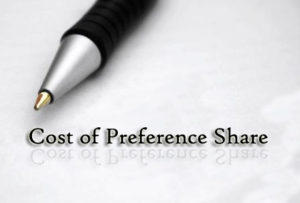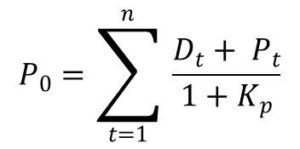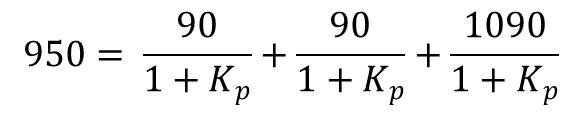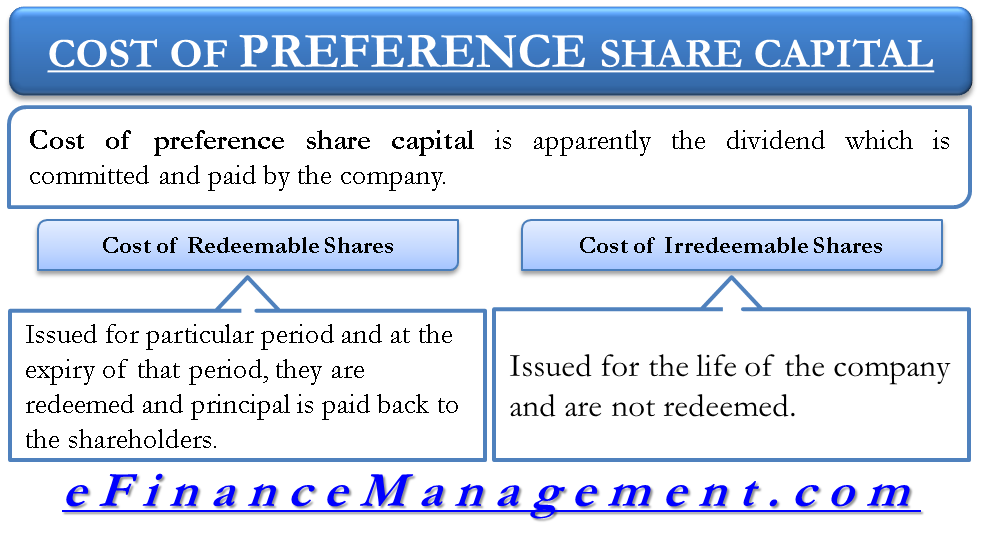# Cost of Preference Share Capital

The cost of preference share capital is apparently the dividend which is committed and paid by the company. This cost is not relevant for project evaluation because this is not the cost at which further capital can be obtained. To find out the cost of acquiring the marginal cost, we will be finding the yield on the preference share based on the current market value of the preference share.

The preference share is issued at a stated rate of dividend on the face value of the share. Although the dividend is not mandatory and it does not create legal obligation like debt, it has the preference of payment over equity for dividend payment and distribution of assets at the time of liquidation. Therefore, without paying the dividend to preference shares, they cannot pay anything to equity shares. In that scenario, management normally tries to pay a regular dividend to the preference shareholders.

Broadly, preference shares can be of two types – Redeemable and Irredeemable.

##Cost of Redeemable Preference Shares

These shares are issued for a particular period and at the expiry of that period, they are redeemed and principal is paid back to the preference shareholders. The characteristics are very similar to debt and therefore the calculations will be similar too. For finding cost of redeemable preference shares, following formula can be used.Cost of Preference Share Formula

Here, preference share is traded at say P0 with dividend payments ‘D’ and principal repayment ‘P’. The cost of debt is designated by Kp. Kcan be determined by solving above equation.

## Explanation of cost of redeemable preference capital with example:

For example, a firm had on the balance sheet, a 9% preference stock which matures after 3 years. The face value is 1000. Putting the formula when current market price of the debenture is 950, we get,Solving the above equation, we will get 11.05%. This is the cost of preference share capital. In the case of debt, it would have required further adjustment with respect to tax because debt enjoys tax shield. Preference dividend is paid out of profits and not treated as an expense for the company. Rather it is called profit distribution.## Cost of Irredeemable Preference Shares

These shares are issued for the life of the company and are not redeemed. Cost of irredeemable preference shares can be calculated as follows:

Here, preference share is traded at say P0 with dividend payments ‘D’. The cost of debt is designated by Kp. Kcan be determined by solving above equation.

## Explanation of cost of irredeemable preference capital with example:

For example, a firm issued a 10% preference stock of \$1000 which has a current market price of \$900. Cost can be calculated as below:

Kp = 100/900

Solving the above equation, we will get 11.11%. This is the cost of redeemable preference share capital.

Last updated on : March 25th, 2019
1.ASA
2.Meenakshi